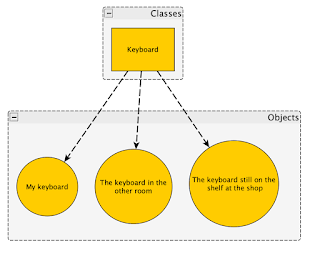## Friday, 8 April 2016

### Project 6: Marching cubes algorithm and metaballs.

The marching cubes algorithm is a way of creating polygons from volumes of data. The data it works on is varied such as 1 / R^2 (the one used to produce metaballs) and a noise function like perlin noise.

My implementation of marching cubes in a nutshell is initially the scene is split into a 3d grid of voxels, then at the corners and center of each voxel an isovalue is calculated (to form the isosurface).
Then using the comparison between the isovalue at each vertex with a threshold value, a table of indices which tell you which edges have been intersected and how each edge is connected to form the triangle facets is referenced.

To generate metaballs from this technique, i used the function 1 / distance^2 to calculate the isovalues at vertices.

Calculating the normal at each triangle facet was done through assuming that each point charge in the scene was a sphere, and the triangle was sitting on the surface of each sphere, individually taking the normals each time. I then took the average of these normals and divided them by the isovalue at the triangles centre. This worked because each charge contributes Q to the triangle, and since the sum of all Q's is equal to the threshold value (or near enough) this allowed me to use it like a weighted average.

--- WARNING AUDIO IN VIDEOS (sorry i forgot) ---

A cool feature of this is that the number of voxels in the grid determines how detailed the object is, here is a scene with 14608 triangles generated (normals enabled):

3416 triangles (no normals):

1384 triangles (no normals):

Heres one showing 2 charges close:

### The difference between class and object.

The difference between class and object is subtle and misunderstood frequently. It is important to understand the difference in order to wr...